# Catapult Energy Conservation

• B
GopherTv
TL;DR Summary
Im trying to calculate the velocity of a projectile on a catapult at different levels of stretch in the spring.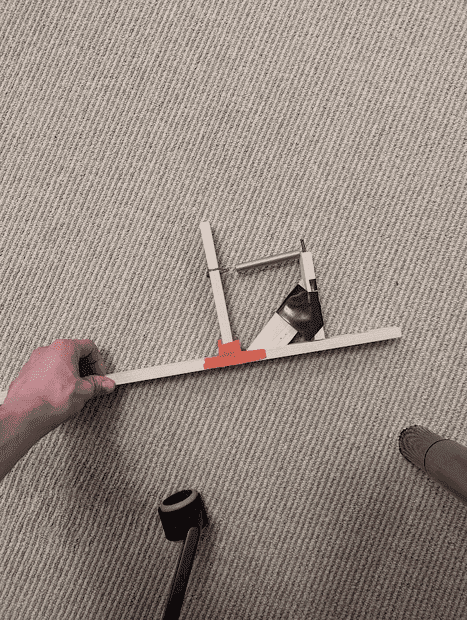This is the catapult.
At equalibrium the spring is 0.09 meters in length.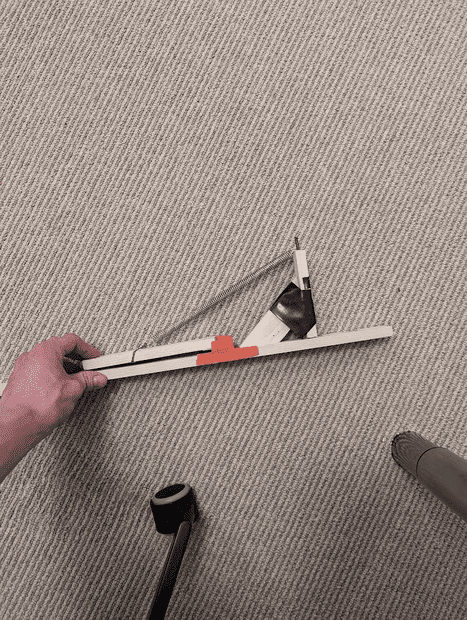When its fully stretched out its 0.225 meters long and I place a rock (0.205 kg) close to where my finger is on the catapult.

The catapult starts with this much energy because 1/2 * k * x^2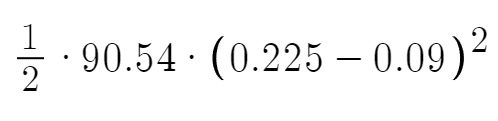90.54 is the spring constant which I got already, 0.225 is the length of the spring and i subtract 0.09 to get the stretch from equalibrium for x.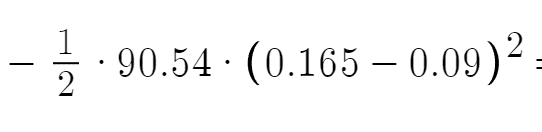i then subtract this because the rock slides off the lever arm before it can fully extend, 0.165 is just a random number i picked for the length of the spring when the rock slides off
.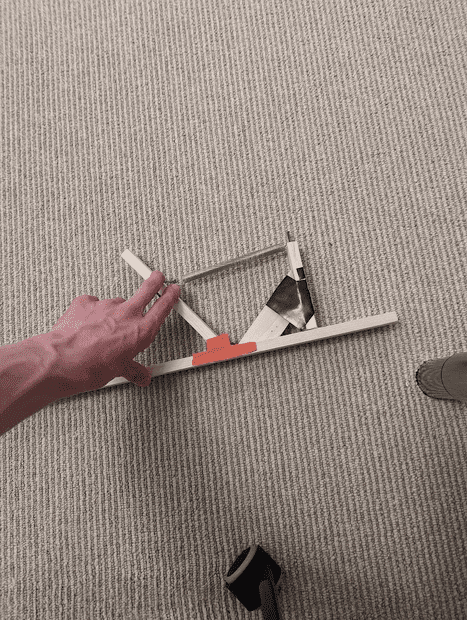We have this: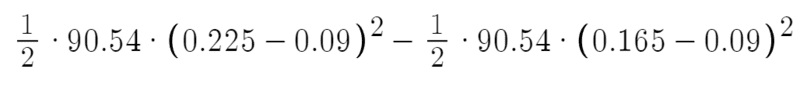This energy goes to 4 things, rotational energy of the lever arm, rotational energy of the rock (since its moving in a circular motion), the gravitational potential energy gained in the rock from it's center of mass now being heigher in the air, and the same for the lever arm, it also gains potential energy.

omega for both the arm and rock will be the same since the angular velocity is same.
subscript 1 is for the lever arm; 2 is for the rock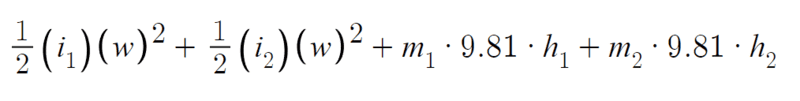the rotational inertia for the lever arm will be 1/3*m*r^2
and for the rock it will be mr^2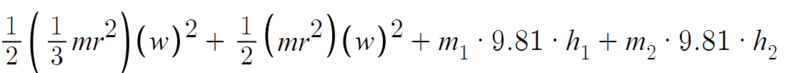GopherTv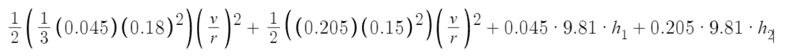I plugged in some numbers, the mass of the lever arm was 0.045 kg and its length was 0.18 meters

The mass of the rock I am launching was 0.205 kg and its distance from the pivot was 0.15 meters

GopherTv
I didn't exactly ask a question when posting, but i wanted to know if my math makes sence. i hope it does